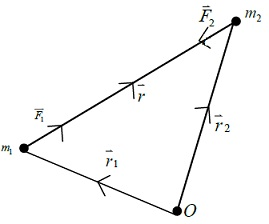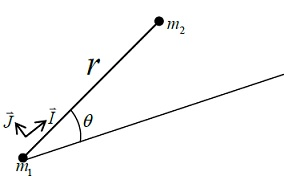#### Satellite Orbits in the Two-Body System, Physics tutorial

Concept of Two-Body Problem:

In practice, there are several investigations which involve more than two bodies. For example, let a hypothetical satellite placed in orbit between the earth and mars. The earth, the sun and mars apply forces on satellite. Therefore, four bodies (Earth, Sun, Mars and satellite itself) are engaged. This is no longer a two-body problem. It is a four-body problem. Luckily, it is frequently allowable to split many-body problem in numerous two-body problems. This technique makes it feasible for approximate values of parameters involved to be computed with relative ease. For example, four-body problem given above can be split in 3 different two-body problems comprising of (a) two body problem involving the earth and the satellite only (b) two-body problem involving sun and the satellite only (c) a two-body problem involving mars and the satellite only.

When satellite is under main attraction of the earth, the two-body problem involving the earth and the satellite becomes about valid. Similar statement holds for each of the remaining two-body problems. Though, in carry-over region where earth and sun attract the satellite with the similar order of magnitude of force, three-body treatment becomes essential. Similar assertion also holds in carry-over region where mars and sun attract the satellite with about the same force. Luckily, these carry-over regions constitute only small fraction of total journey of satellite in its orbit. As a result, much calculation time is saved using this technique.

Solution to Two-Body Problem:

Let two bodies of masses m1 and m2 separated by the linear displacement r. Newton's second law of motion defines that

F = d(mv)/dt or F = md2r/dt2 or md2r/dt2 = ∑i=1kFiForce of attraction, F1, on m1 is directed along vector r. Force of attraction, F2, on m2 is in opposite direction.

By Newton's third law,

F1= -F2

Also, by Newton's law of gravitation,

F1= Gm1m2/r2(r/r)

Again

F2 = -G(m1m2/r2)(r/r)

Let vectors r1 and r2be directed from some fixed reference point O to particles of masses m1 and m2, respectively.

Now,

F = m(d2r1/dt2)

so that

F1 = m1(d2r1/dt2) and F2 = m2(d2r2/dt2)

Equation (1) can then be rewritten as

m1(d2r1/dt2) = (Gm1m2/r2)(r/r)

Equation (2) can also be rewritten as

m2(d2r2/dt2) = (Gm1m2/r2)(r/r)

m1(d2r2/dt2) + m2((d2r2/dt2)) = 0

by definition, the centre-of-mass C of the system of masses m1 and m2 is given by MR = m1r1 + m2r2 where M = m1 + m2 and R is the position vector of C Hence, by Eq.(8), you get

M(dR/dt) = a Also by equation we get

MR= at + b

These two relations show that centre of mass of the system moves with constant velocity (i.e., with constant speed in straight line).

Angular Momentum Integral:

Equations may be written as

d2r/dt2 = Gm2(r/r3) and d2r2/dt2 = -Gm1(r/r3) respectively

After solving equation gives

d2(r1 - r2)/dt2 = G(m1 + m2)(r/r3) But from figure

r1 - r2 = -r Hence d2r/dt2 + μr/r3 = 0

where μ = G(m1 + m2)

Taking the vector product of r→ with Equation you obtain

rxd2r/dt2 + μr/r3 x r = 0

i.e.

Integrating, we have rx dr/dt = hwhere h is constant vector.

Equation is angular momentum integral. Now, as h is constant, it should point in one direction or all values of t. Therefore, motion of one body about other should lie in plane defined by th direction of h.

Mathematical form of Kepler's Second Law:

If polar coordinates r and θ are taken in this plane velocity component along radius vector joining m1 to m2 is r^. Also, perpendicular component of radius vector is rθ^, where dot replaces d/dt.By defining I and J as the unit vectors along and perpendicular to the radius vector, the resultant velocity r→^ becomes

r^ = Ir. + J.

Therefore, using Equations we can easily verify each of the given steps:

rx dr/dt = h

rx r^ = h

rx(Ir. + J.) = h

Irx(Ir. + J.) = h

(IrxI)r.+(Ir x J)rθ. = h

0 + (Kr)rθ. = h

Thus Kr2θ. = h

We may thus write:

r2θ. = h

Here constant h is twice the rate at which radius vector defines the area. This is mathematical form of Kepler's second law. For the circular orbit, rate of change of area may, of course, be evaluated as follows:

A = πr2

dA/dt = dA/dr x dr/dt

2πrdr/dt => 2πrr.

Energy Conservation Equation of System:

If scalar product of r. with equation is now taken, we get

r..d2r/dt2 + μr..r/r3 = 0 i.e. r..r.. + μ/r3 r..r = 0

This may be integrated to provide

1/2r..r.-μ/r = c

Here C is a constant v.

I.e., 1/2v2 - μ/r = c

Here v is velocity.

Equation is energy conservation equation of the system. Quantity C isn't the total energy of system. Though, quantity 1/2v2 is associated to kinetic energy whereas quantity -μ/r is associated to potential energy of the system.

Satellite Obits in Two-Body System:

The components of acceleration along and perpendicular to radius vector are

r.. - rθ.2 and 1/2(d/dt)(r2θ.) respectively. Equation can be written as:

I(r.. - rθ.2) + J[(1/r)(d/dt)(r2θ.)] + (μ/r3)(Ir) = 0

Equating coefficients of vectors, we get:

r.. - rθ.2 = -μ/r2 and (1/r)(d/dt)(rθ.2) = 0

Integration of equation provides angular momentum integral

r2θ. = h

Substituting u = 1/r and eliminating time t between equations we get:

d2u/dt2 + u = μ/h2

General solution of equation is as follows:

u = μ/h2 + Acos(θ-ω)

Here A and ω are two constants of integration. Reintroducing r in equation it becomes:

1/r = μ/h2 + Acos(θ-ω) or

r = [1/(μ/h2 + Acos(θ-ω))] or

r = [(h2/μ)/1-(Ah2/μ)cos(θ-ω)]

But, generally, polar equation of the conic section may be written as:

r = P/(1 + ecos(θ-ω)) so that p = h2/μ and e = Ah2

Therefore, solution of two-body problem is a conic section. It comprises Kepler's first law as special case. Particularly, orbit of a satellite about earth is categorized by value of eccentricity e. Four cases to be remembered are:

i) For 0 < e < 1, orbit is ellipse.

ii) For e = 1, orbit is parabola.

iii) For e >1, orbit is hyperbola.

iv) For e = 0, orbit is circle

Tutorsglobe: A way to secure high grade in your curriculum (Online Tutoring)